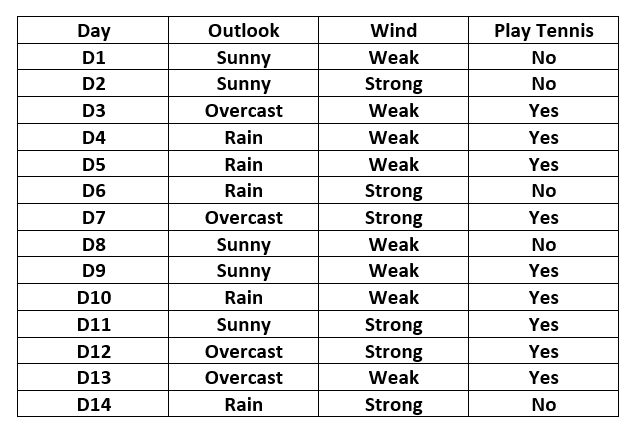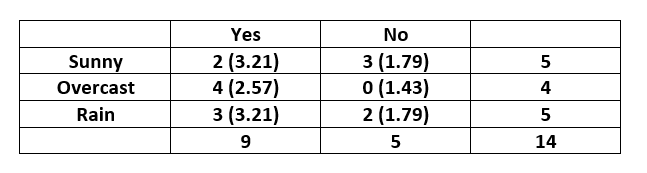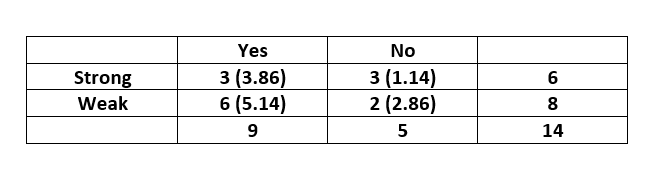# Chi-Square Test for Feature Selection – Mathematical Explanation

One of the primary tasks involved in any supervised Machine Learning venture is to select the best features from the given dataset to obtain the best results. One way to select these features is the Chi-Square Test.

Mathematically, a Chi-Square test is done on two distributions two determine the level of similarity of their respective variances. In its null hypothesis, it assumes that the given distributions are independent. This test thus can be used to determine the best features for a given dataset by determining the features on which the output class label is most dependent on. For each feature in the dataset, theis calculated and then ordered in descending order according to thevalue. The higher the value of, the more dependent the output label is on the feature and higher the importance the feature has on determining the output.

Let the feature in question have m attribute values and the output have k class labels. Then the value ofis given by the following expression:-where– Observed frequency– Expected frequency

For each feature, a contingency table is created with m rows and k columns. Each cell (i,j) denotes the number of rows having attribute feature as i and class label as k. Thus each cell in this table denotes the observed frequency. To calculate the expected frequency for each cell, first the proportion of the feature value in the total dataset is calculated and then it is multiplied by the total number of the current class label.

Solved Example:

Consider the following table:-Here the output variable is the column named “PlayTennis” which determines whether tennis was played on the given day given the weather conditions.

The contingency table for the feature “Outlook” is constructed as below:-Note that the expected value for each cell is given inside the parenthesis.

The expected value for the cell (Sunny,Yes) is calculated asand similarly for others.

Thevalue is calculated as below:-The contingency table for the feature “Wind” is constructed as below:-Thevalue is calculated as below:-On comparing the two scores, we can conclude that the feature “Wind” is more important to determine the output than the feature “Outlook”.

My Personal Notes arrow_drop_upCheck out this Author's contributed articles.

If you like GeeksforGeeks and would like to contribute, you can also write an article using contribute.geeksforgeeks.org or mail your article to contribute@geeksforgeeks.org. See your article appearing on the GeeksforGeeks main page and help other Geeks.

Please Improve this article if you find anything incorrect by clicking on the "Improve Article" button below.

Improved By : ManasChhabra2

Article Tags :
Practice Tags :

3

Please write to us at contribute@geeksforgeeks.org to report any issue with the above content.